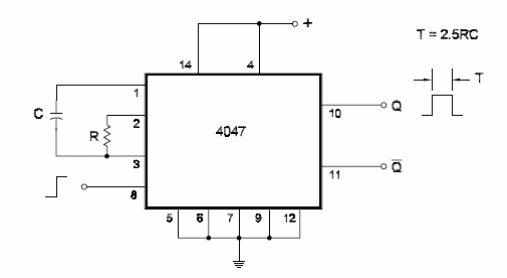### DatasheetsN° of component

## Monostable Using the 4047 (I) (CB134E)

The 4047 is a monostable/astable CMOS multivibrator. The basic con- figuration is triggered by the positive transition of the input signal is can be seen in the figure bellow. The time constant for this circuit is 2.4 x R x C. The circuit has complementary outputs. When triggered, 0 goes to the “1" logic level at the same time as 0/ goes to the “0" logic level. R must be in the range of 1 k ohm and 10 M ohm, and the maximum value recommended for C is 100 pF. If R is 22 k ohm and C is 10 pF, then the amount of time for this circuit to be on is 2 us. If R is 220 k and C is 1000 pF, then the amount of time for this circuit to be on is 550 us.Monostable Using the 4047 (I)

Datasheet of 4047